Check If a Cell contain Image/Picture

This post will guide you how to check if a specific cell contains an image or picture in excel. Is it possible to check if a cell contains an image. This post will guide you how to define a User Defined Function to determine if an image exists in a specified cell. Or how to use VBA Macro code to check if a cell contains an image.

The simplest method is to create a user defined function to check if a cell contains an image file, and return 1 if image exists in cell. Otherwise, returns 0. You can follow these steps:

#1 open your excel workbook and then click on “Visual Basic” command under DEVELOPER Tab, or just press “ALT+F11” shortcut.#2 then the “Visual Basic Editor” window will appear.

#3 click “Insert” ->”Module” to create a new module#4 paste the below VBA code into the code window. Then clicking “Save” button.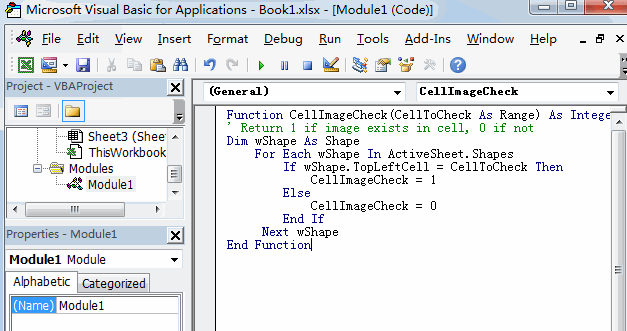Function CellImageCheck(CellToCheck As Range) As Integer
' Return 1 if image exists in cell, 0 if not
Dim wShape As Shape
For Each wShape In ActiveSheet.Shapes
If wShape.TopLeftCell = CellToCheck Then
CellImageCheck = 1
Else
CellImageCheck = 0
End If
Next wShape
End Function

#5 back to the current worksheet, try to enter the below formula in Cell D6.

=CellImageCheck(B6)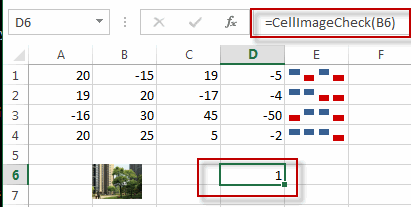You can also use the following VBA code to check if a cell contains an image.  You just need to repeat the above 1-3 steps firstly, then continue to do the following steps:

#1 paste the below VBA code into the code window. Then clicking “Save” button.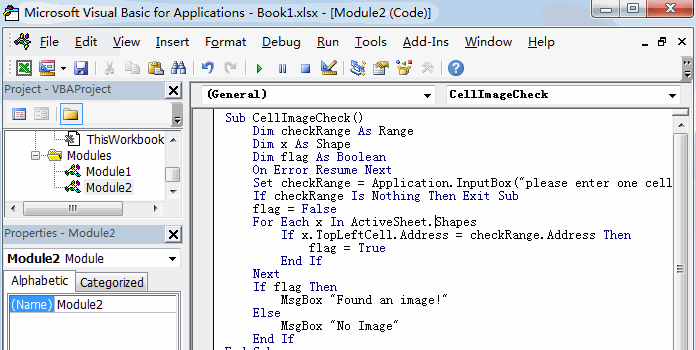Sub CellImageCheck()
Dim checkRange As Range
Dim x As Shape
Dim flag As Boolean
On Error Resume Next
Set checkRange = Application.InputBox("please enter one cell that you want to check", "CellImageCheck", Selection.Address, , , , , 8)
If checkRange Is Nothing Then Exit Sub
flag = False
For Each x In ActiveSheet.Shapes
flag = True
End If
Next
If flag Then
MsgBox "Found an image!"
Else
MsgBox "No Image"
End If
End Sub

#2 back to the current worksheet, then run the above excel macro. Click Run button.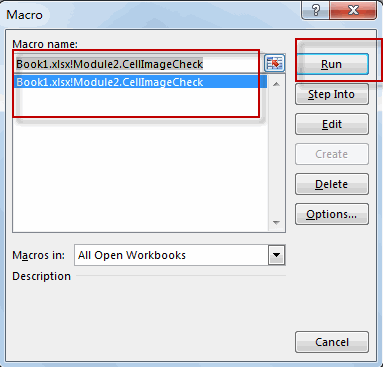#3 select one cell that you want to check, such as: B6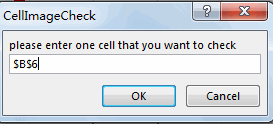#4 Let’s see the result: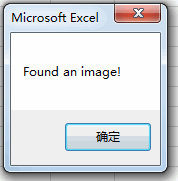Related Posts

VBA Macro For VLOOKUP From Another Sheet

In the previous post, you should know that how to fix or remove the #N/A error when using VLOOKUP formula to lookup value from another sheet. And this post will show you how to use VBA code to vlookup data ...

How To Insert Comments in Protected Worksheet in Excel

This post will show you how to allow comments in a protected worksheet in Excel. You can easily to insert comments into cells in a normal worksheet in Excel, but if want to insert a comment in a worksheet that ...

How To Convert Text to Upper Cases(Using VBA) in Excel

This post will show you how to switch from lower case to upper case in Excel. and I am going to show you two different ways of converting text to upper cases using formula or VBA macro in Excel 2013,Excel ...

How To Hide Every Other Row in Excel (Using VBA)

This post will show you how to hide alternate rows or columns in Excel or how to hide every third, fourth, fifth row or column in Excel. If you want to hide every other row in your current worksheet, how ...

How to Disable the Save As Prompt in Excel

This post will show you how to use a VBA Macro to save an Excel file and overwrite any existing file without a prompt so that you are going to get the little window that says file already exists do ...

How to Count Cells that Contain even or odd numbers in Excel

This post will guide you how to count the number of cells that contain odd or even numbers within a range of cells using a formula in Excel 2013/2016.How do I count cells that contain odd numbers through the use ...

How to Count Cells that Contain negative Numbers in Excel

This post will guide you how to count the number of cells that contain negative numbers within a range of cells using a formula in Excel 2013/2016.You can count the number of negative numbers in your data using easy functions ...

How to Count Cells Are Not Blank or Empty in Excel

This post will guide you how to count cells that are not blank or empty in a given range cells using a formula in Excel 2013/2016.How do I count the number of cells that are not blank in a particular ...

How to Count Cells Less Than a Specific Value in Excel

This post will guide you how to count the number of cells less than a particular numeric value in a given range cells using a formula in Excel 2013/2016. How do I count cells that are less than a specific ...

How to Count Cells Greater Than a Specific Value in Excel

This post will guide you how to count the number of cells greater than a particular numeric value in a given range cells using a formula in Excel 2013/2016. How do I count cells that are greater than a specific ...

Sidebar